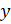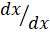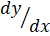### INTEGRATION

Mathematics SSS 3 Second Term

WEEK 1

INTEGRATION

Performance Objectives

Student should be able to:

1. Understand integration as the reverse of differentiation.
2. Understand the various techniques of integration.
3. Apply these techniques to solve problems.

Content

In integration, we wish to find back the function ywhen its differential coefficient dxdxis given. Therefore the process of finding ywhen dydxis given is called integration. So in general if

dydx=g View More